# Fraction Calculator

Fraction calculator allows you to do addition, subtraction, division, and multiplication of simple and mixed fractions online.

 + - × ÷
 =
##### More Useful Tools
Decimal to Fraction Calculator Quadratic Formula Calculator

## What is Fraction Calculator?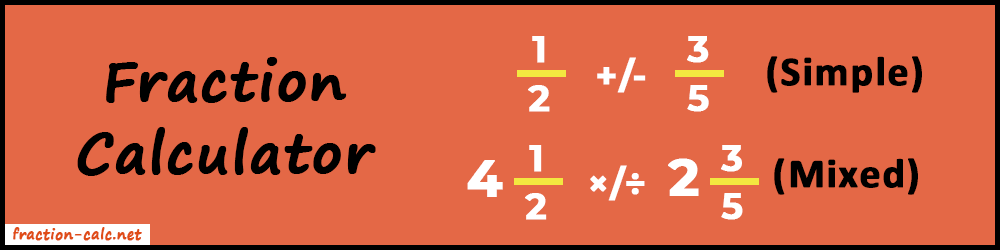The mathematics world doesn't only have integer numbers. There are also fractional and decimal numbers. We use fractions to show a definite portion of the whole.

It's very difficult to represent or quantify partial amounts without the use of fractions. We can easily tell that there are 4 books lying on the desk or I am drinking one glass of juice. But we need the help of fractions to quantify amounts like half or two-thirds of a glass of juice. Hence, we can easily tell a 1/2 or 2/3 glass of juice.

The fraction calculator is very helpful in real life. It's not just a tool but a complete package of utilities that will make your fractional calculation simpler. One special thing about it is that it supports two kinds of fraction calculation. "Simple and Mixed". So, you can toggle between them whichever you want to use. Accordingly, the input boxes will appear where you need to enter your values.

Also, in this article, we will learn about fractions, and how to add, subtract, multiply, and divide two fractions manually. Lastly, we will see the various uses of our calculator.

### Fraction Number

In mathematics, we use fractions to represent or quantify some parts out of a whole quantity or number.

For Example:

2
4

Let's understand it with a real-life example.

Suppose you have a cake that has 5 slices. Now, if you want to represent one slice of the whole cake then you can tell as 1/5 of the cake. It means you have 20% part out of the whole cake. Combining all these individual slices will give the full cake.

20% x 5 = 100% or 1/5 x 5 = 1

In addition, if you give one piece to your friend and one to your brother. Then what is the amount of cake you are left with?

Let's figure out.

Full Cake = 1
Total number of pieces = 5
One slice = 1/5
Two slices are given = 1/5 + 1/5 = 2/5 or 1/5 x 2 = 2/5
Remaining slices = 1 – 2/5 = 3/5

Therefore, you are left with a 3/5 amount of cake.

You can see, this calculation is as simple as eating a slice of cake. But you will not always encounter the same standard of problems. In all that case, the Fraction Calculator is the only tool that will be useful for you. Even more, you can easily evaluate simple to complex fractional operations within a second.

#### Numerator and Denominator

In fractions, you have to deal with numerators and denominators. It is important to know what they are. So, let's learn about these two things in detail.

First of all, let's take an example.

3 → Numerator
5 → Denominator

The numerator is the value of the amount of portion out of the whole. Whereas, the denominator represents the whole value. Let's understand it using the blank paper example.

There are 5 parts of the blank paper, So, 5 is the total amount or number. Now, one individual part means 1/5 of the full paper.

Here, 1 is the value of the amount of portion out the whole paper(i.e. 1 part). So, it's placed in the numerator.

1 piece of paper is out of the 5 parts. Therefore, 5 becomes the denominator. As it is the whole value.

When the denominators are same then fractional addition is very easy. Just sum up the numerators. Whereas denominator remains same.

For example:

 3 7
+
 2 7
=
 5 7

In the case when denominators are different we have to find the Least Common Multiple (LCM) of the denominators.

So, let's take an example and know how to find LCM of denominators.

 x y
+
 p q
=
 xq + py yq
 2 3
+
 1 4
=
 8 + 3 12
=
 11 12

#### Subtraction

Just like addition, if the denominators are the same then subtraction also becomes easier. Just subtract the numerators and the denominator remains the same.

 3 7
-
 2 7
=
 1 7

Here also, we need to determine the LCM of the denominators if they are different.

##### Subtract 5/3 with 2/4
 x y
-
 p q
=
 xq - py yq
 5 3
-
 2 4
=
 20 - 6 12
=
 14 12
=
 7 6

#### Multiplication

Fractional multiplication is very straightforward unlike addition and subtraction. Look at this formula below to multiply any two fractions.

 x y
×
 p q
=
 xp yq

Multiply the numerator with the numerator and denominator with the denominator.

##### Multiply 3/5 with 4/7
 3 5
×
 4 7
=
 3×4 5×7
=
 12 35

#### Division

Dividing fractions is similar to multiplying fractions but with a little twist. We have to do the cross-multiplication.

##### Divide 3/5 with 2/6
 x y
÷
 p q
=
 xq py
 3 5
÷
 2 6
=
 3×6 5×2
=
 18 10
=
 9 5

As the complexity of the problem increases it will take more time to solve the equation. So, in that case, use our Fraction Calculator to make this complex work easy and fast.

#### Features of Fraction Calculator

• Fast: Think yourself how much time would you take to solve a complex problem on your own. Now, how much time will this calculator take? Surely, less than a second! So, it is more efficient and useful.
• Multiple Operations: It supports almost all arithmetic operations. Such as addition, subtraction, multiplication, and division. Also, it allows doing these operations on simple and mixed fractions.
• Accurate: It's human nature to commit mistakes. But no one knows how much that mistake can cost. Therefore, not taking a risk is valuable advice. This calculator gives 100% accurate answers. Because it works on javascript programming algorithms. So, there is no chance for wrong output.
• Convenient: It is an online utility web app that can be used anytime anywhere. Also, you can use it on your handy devices like mobile or iPad. Because we have designed it mobile and iPad responsive.

### How to use Fraction Calculator?

First of all, you need a smart device with an active internet connection. Because our tool is online and you can only access it if you have the internet on your phone or laptop. After that, open the Fraction Calculator and follow the instructions below.

##### 1. Select Fraction Type

Our tool supports two types of fraction calculation.

1. Simple Fractions
2. Mixed Fraction

Yes, our supports both types of calculation. So, you don't have to find another calculator to do the mixed number calculation.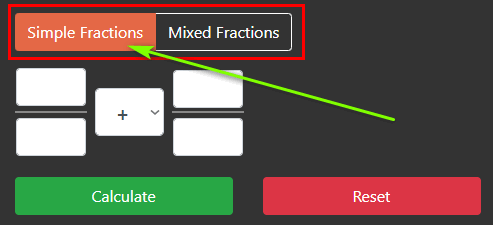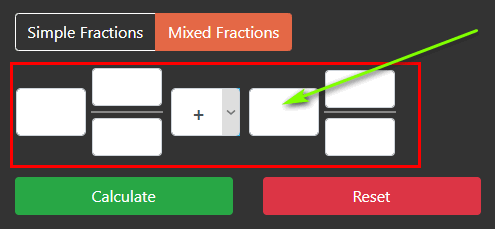##### 2. Enter Fraction Values

The next step is to enter values inside the empty fields. If you choose simple one then you have to enter 4 values. But if you choose the mixed one then you need to enter 6 values.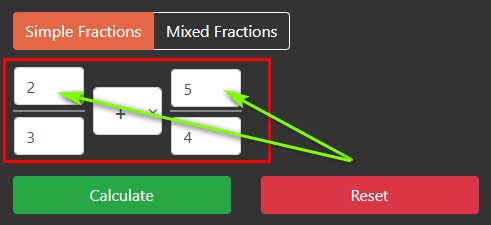##### 3. Select Operation

Our tool supports four operations to perform.

2. Subtraction
3. Multiplication
4. Division

So, choose the operation that you need to perform between two fractions values.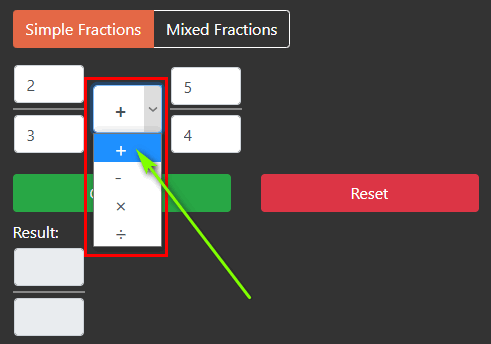##### 4. Start Calculation

Lastly, click on the "Calculate" button to start the calculation process. Also, you can use "Reset" button for recalculation.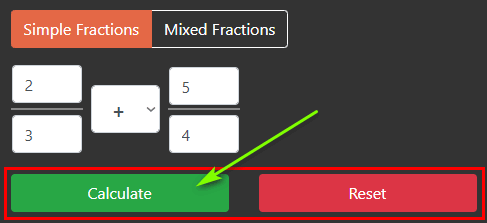##### 5. Get Output with Calculation

As a result, you will get the final output. Also, you will get the full calculation with entered values at the bottom.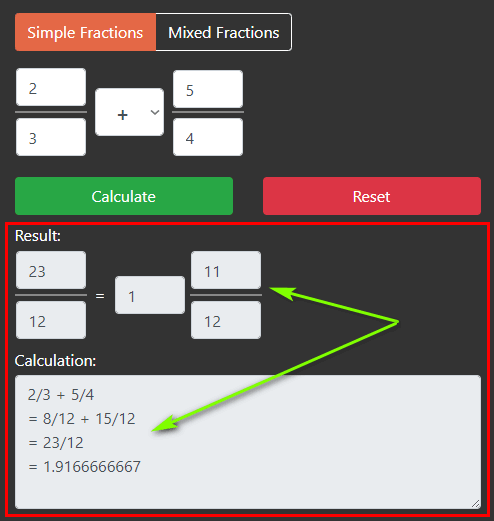### Conclusion

Above all was about the fraction calculator. Make your fraction calculations easier with our online tool. It can help you with a lot of things. Maybe in checking your homework, calculating offers or prices, determining different sorts of amounts, and much more.